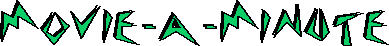Main      Site Guide# Wild Things

## Directed by John McNaughton

### 1998

##### Ultra-Condensed by Samuel Stoddard

(There is a PLOT TWIST. Then there is a PLOT TWIST. Then there is a PLOT TWIST. Then there is a PLOT TWIST. Then there is a PLOT TWIST. Then there is a PLOT TWIST. Then there is a PLOT TWIST.)

THE END

(Then there is a PLOT TWIST. Then there is a PLOT TWIST. Then there is a PLOT TWIST.)